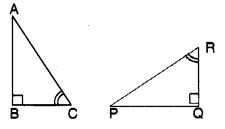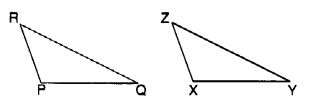# CBSEtips.in

## Thursday, 25 February 2021

### CBSE Class 7 Maths - MCQ and Online Tests - Unit 7 - Congruence of Triangles

#### CBSE Class 7 Maths – MCQ and Online Tests – Unit 7 – Congruence of Triangles

Every year CBSE students attend Annual Assessment exams for 6,7,8,9,11th standards. These exams are very competitive to all the students. So our website provides online tests for all the 6,7,8,9,11th standards’ subjects. These tests are also very effective and useful for those who preparing for any competitive exams like Olympiad etc. It can boost their preparation level and confidence level by attempting these chapter wise online tests.

These online tests are based on latest CBSE syllabus. While attempting these, our students can identify their weak lessons and continuously practice those lessons for attaining high marks. It also helps to revise the NCERT textbooks thoroughly

#### CBSE Class 7 Maths – MCQ and Online Tests – Unit 7 – Congruence of Triangles

Question 1.
If ? ABC = ? PQR, then $$\overline { CA }$$ corresponds to
(a) $$\overline { PQ }$$
(b) $$\overline { QR }$$
(c) $$\overline { RP }$$
(d) none of these

Answer: (c) $$\overline { RP }$$

Question 2.
We want to show that ? ART = ? PEN and we have to use SSS criterion. We have AR = PE and RT = EN. What more we need to show?
(a) AT = PN
(b) AT = PE
(c) AT = EN
(d) none of these

Answer: (a) AT = PN

Question 3.
If ? ABC = ? PQR, then ?A corresponds to
(a) ?P
(b) ?Q
(c) ?R
(d) none of these

Question 4.
For two given triangles ABC and PQR, how many matchings are possible?
(a) 2
(b) 4
(c) 6
(d) 3

Hint:
ABC ? PQR, ABC ? PRQ,
ABC ? QRP, ABC ? QPR,
ABC ? RPQ, ABC ? RQP.

Question 5.
The symbol for congruence is
(a) =
(b) ?
(c) ?
(d) =

Question 6.
The symbol for correspondence is
(a) =
(b) ?
(c) =
(d) ?

Question 7.
‘Under a given correspondence, two triangles are congruent if the three sides of the one are equal to the three corresponding sides of the other.’
The above is known as
(a) SSS congruence of two triangles
(b) SAS congruence of two triangles
(c) ASA congruence of two triangles
(d) RHS congruence of two right-angled triangles

Answer: (a) SSS congruence of two triangles

Question 8.
‘Under a given correspondence, two triangles are congruent if two sides and the angle included between them in one of the triangles are equal to the corresponding sides and the angle included between them of the other triangle.’
The above is known as
(a) SSS congruence of two triangles
(b) SAS congruence of two triangles
(c) ASA congruence of two triangles
(d) RHS congruence of two right-angled triangles

Answer: (b) SAS congruence of two triangles

Question 9.
If ? ABC = ? PQR, then $$\overline { AB }$$ corresponds to
(a) $$\overline { PQ }$$
(b) $$\overline { QR }$$
(c) $$\overline { RP }$$
(d) none of these

Answer: (a) $$\overline { PQ }$$

Question 10.
If ? ABC = ? PQR, then $$\overline { BC }$$ corresponds to
(a) $$\overline { PQ }$$
(b) $$\overline { QR }$$
(c) $$\overline { RP }$$
(d) none of these

Answer: (b) $$\overline { QR }$$

Question 11.
If ? ABC = ? PQR, then ?B corresponds to
(a) ?P
(b) ?Q
(c) ?R
(d) none of these

Question 12.
If ? ABC= ? PQR, then ?C corresponds to
(a) ?P
(b) ?Q
(c) ?R
(d) none of these

Question 13.
‘Under a given correspondence, two triangles are congruent if two angles and the side included between them in one of the triangles are equal to the corresponding angles and the side included between them of the other triangle.’
The above is known as
(?) SSS congruence of two triangles
(b) SAS congruence of two triangles
(c) ASA congruence of two triangles
(d) RHS congruence of two right-angled triangles

Answer: (c) ASA congruence of two triangles

Question 14.
‘Under a given correspondence, two right-angled triangles are congruent if the hypotenuse and a leg of one of the triangles are equal to the hypotenuse and the corresponding leg of the other triangle.’
The above is known as
(?) SSS congruence of two triangles
(b) SAS congruence of two triangles
(c) ASA congruence of two triangles
(d) RHS congruence of two right-angled triangles

Answer: (d) RHS congruence of two right-angled triangles

Question 15.
We want to show that ? ART = ? PEN. We have to use SAS criterion. We have ?T = ?N, RT = EN. What more we need to show?
(a) PN = AT
(b) PN = AR
(c) PN = RT
(d) None of these

Answer: (a) PN = AT

Question 16.
If ? ABC and ? PQR are to be congruent, name one additional pair of corresponding parts(a) BC = QR
(b) BC = PQ
(c) BC = PR
(d) none of these

Answer: (a) BC = QR

Question 17.
By which congruence, is ? ABC = ? FED?(a) SSS
(b) SAS
(c) ASA
(d) RHS

Hint:
?B = ?E, BC = ED, ?D = ?C
(By angle sum property of a triangle).

Question 18.
Which congruence criterion do you use in the following?Given : ZX = RP
RQ = ZY
? PRQ = ? XZY
So, ? PRQ = ? XYZ
(a) SSS
(b) SAS
(c) ASA
(d) RHS

Question 19.
Which congruence criterion do you use in the following?(a) SSS
(b) SAS
(c) ASA
(d) RHS

Question 20.
Which congruence criterion do you use in the following?(a) SSS
(b) SAS
(c) ASA
(d) RHS

Question 21.
In the following figure, the two triangles are congruent. The corresponding parts are marked. We can write ? RAT = ?(a) ? WON
(b) ? WNO
(c) ? OWN
(d) ? ONW

Answer: (a) ? WON

Question 22.
Complete the congruence statement ? BCA = ?(a) ? BTA
(b) ? BAT
(c) ? ABT
(d) ? ATB

Answer: (a) ? BTA

Question 23.
Complete the congruence statement ? QRS(a) ? TPQ
(b) ? TQP
(c) ? QTP
(d) ? QPT

Answer: (a) ? TPQ

Question 24.
We want to show that ? ART = ? PEN. We have to use ASA criterion. We have AT = PN, ?A = ?P. What more we need to show?
(a) ?T = ?N
(b) ?T = ?E
(c) ?T = ?P
(d) None of these

Answer: (a) ?T = ?N

Question 25.
Which congruence criterion do you use in the following?Given AC = DF
AB = DE
BC = EF
So, ? ABC ? ? DEF
(a) SSS
(b) SAS
(c) ASA
(d) RHS